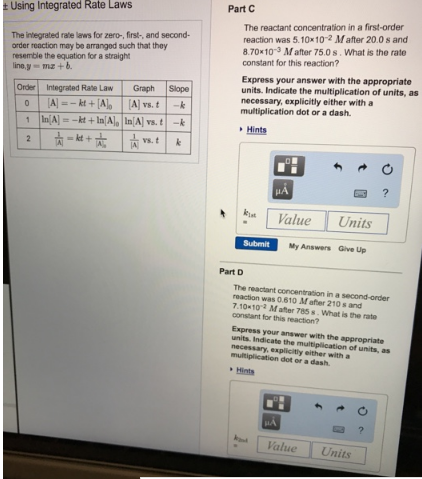# Problem: The integrated rate laws for zero-, first-, and second-order reaction may be arranged such that they resemble the equation for a straight line, y = mx + b. Part CThe reactant concentration in a first-order reaction was 5.10 x 10-2 M after 20.0 S and 8.70 x 10-3 M after 75.0 S. What is the rate constant for this reaction? Express your answer with the appropriate units. Indicate the multiplication of units, as necessary, explicitly either with a multiplication dot or a dash. Part D The reactant concentration in a second-order reaction was 0.610 M after 210 s and 7.10 x 10-2 M after 785 S. What is the rate constant for this reaction?Express your answer with the appropriate units. Indicate the multiplication of units, as necessary, explicitly either with a multiplication dot or a dash.

###### FREE Expert Solution
83% (125 ratings)###### Problem Details

The integrated rate laws for zero-, first-, and second-order reaction may be arranged such that they resemble the equation for a straight line, y = mx + b.

Part C
The reactant concentration in a first-order reaction was 5.10 x 10-2 M after 20.0 S and 8.70 x 10-3 M after 75.0 S. What is the rate constant for this reaction?

Express your answer with the appropriate units. Indicate the multiplication of units, as necessary, explicitly either with a multiplication dot or a dash.

Part D

The reactant concentration in a second-order reaction was 0.610 M after 210 s and 7.10 x 10-2 M after 785 S. What is the rate constant for this reaction?

Express your answer with the appropriate units. Indicate the multiplication of units, as necessary, explicitly either with a multiplication dot or a dash.